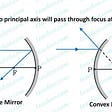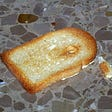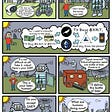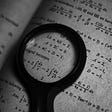# Covariance vs Correlation: Which should you use?

Covariance and correlation, you have probably come across these terms in probability theory and statistics. They both are used to describe a very similar aspect i.e the type of linear relationship between some random variables/ features. But then what are the differences between these terms and which one should you use?

To answer these questions I’ll first start by giving a brief overview of these topics. I’ll be using car specifications as an analogy for better intuition.

Covariance

Covariance signifies the direction of the linear relationship between some random variables i.e if the variables are directly proportionate or inversely proportionate. These directions can be of three types: Positive, Negative, or Neutral.

Covariance can be calculated by the formula:

Where n is the number of data points in the x or y variable. The values can range from -inf to +inf.

When there is a positive covariance, i.e Cov(x, y) > 0, an increase in one variable results in the increase of the other. The given graph illustrates that as the horsepower of a car increases so does its price.

If there is a negative covariance, i.e Cov(x, y) < 0, an increase in one variable results in the decrease of the other. Here you can see that increase in horsepower leads to a decrease in its mileage.

If the covariance is neutral, i.e Cov(x, y) is close to 0, then the change in a variable doesn’t affect the other. Both the variables are independent of each other. You can see that bore(size of each cylinder in an engine) is unaffected by the stroke(distance traveled by a piston).

But, covariance has its limitations. It shows only the direction and not the strength of the relationship.

Strength? By strength, I mean the degree to which the two variables move in relation to each other. You could also say it’s the magnitude of the relationship.

So why is the strength of the linear relationship important?

Suppose you calculate the covariance of:

1) horsepower and price

2) horsepower and curb weight (weight of a car without any passengers or baggage)

Now you want to find the feature which has a stronger relationship with horsepower so that you can remove the other (maybe to reduce the number of features). But the above plots show that both have positive covariance.

So how do you understand which variable has a stronger linear relation with horsepower?

(Note that the value of covariance does not indicate the strength of the relationship. Its value is dependent on the scale/metric of the variable eg. meters, centimeters, kilos. So it depends on the domain.)

Correlation

Correlation describes the direction as well as the strength/magnitude of the relationship of the random variables.

The strength of a linear relationship can be described by taking the variance of the variables into account. So, the correlation of variables x and y can be calculated with the formula:

Unlike covariance, the value of correlation ranges from -1 to +1. The sign indicates the direction of the relation, that is if they are directly or inversely proportionate to each other.

A perfect correlation is represented by a correlation coefficient value of 1, with +1 indicating that there is a perfect positive correlation and -1 indicating that there is a perfect negative correlation. 0 denotes that there is no correlation between the variables.

So, the types of correlation are:

• +1: Positive Correlation
• - 1: Negative Correlation
• 0: No Correlation

As a rule of thumb correlation coefficient between -0.3 and +0.3 is considered weak, while a coefficient less than -0.7 and greater than +0.7 is considered strong.

So finally coming to the above problem, to find the variable more closely correlated to horsepower between the price of the car or its curb weight.

Let’s calculate the correlation between the two sets of variables:

• Correlation(horsepower, price) = 0.81053
• Correlation(horsepower, curb weight) = 0.7580

You can see that both the variables have a positive correlation with horsepower. However, the price has a stronger correlation compared to curb weight.

Conclusion

Both these concepts have a limitation, they are only able to map the linear relationship of random variables. But if you have to choose between covariance or correlation to understand the linear relationship between any given variables, you should go with the latter as it indicates the direction as well as the magnitude of how well the two variables are correlated. Also unlike covariance, its value isn’t affected by the scale or metric of the variables and always normalizes between -1 and +1.

--

--

--

Hey! I am interested in AI, Technology, Statistics, and how AI could impact well-being.

Love podcasts or audiobooks? Learn on the go with our new app.

## Can Astrology Say about Lottery Chance?## The dimension of the Residence Time Distribution function is 1/Time.## How to draw a perfect ray diagram for Mirrors : 4 Best Rules## The laws of improbability## How to Decide the Suitability of Approximation Algorithms?Hey! I am interested in AI, Technology, Statistics, and how AI could impact well-being.

## Bagging vs Boosting in Machine Learning training process## Choosing the Right Evaluation Metric## When It Comes to Clustering Algorithms, Less Is Usually More## Point Estimates, Confidence Intervals, Z-Test vs T-Tests (One sample) [Part 1]